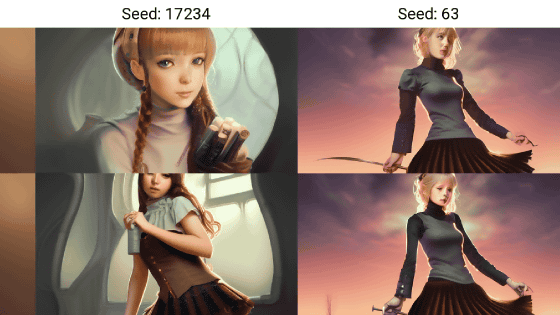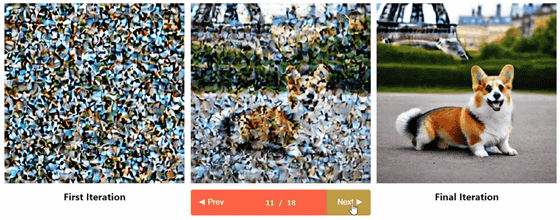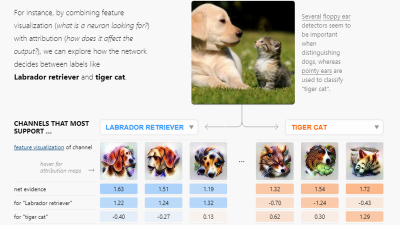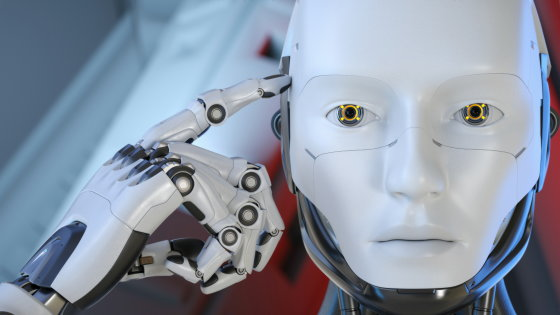# An easy-to-understand illustration of ``how to draw a picture'' that you can understand if you know how to master the image generation AI ``Stable Diffusion''The image generation AI `` Stable Diffusion '', which was released to the public free of charge in August 2022, can generate arbitrary character strings and images by anyone with a CPU equipped with an NVIDIA GPU or an online execution environment such as Google Colaboratory. can be generated. AI Pub, which explains AI on Twitter, explains how such Stable Diffusion generates images.

You can roughly understand how Stable Diffusion generates images by looking at the GIF animation that you can see by clicking the image below.In the first place, 'Diffusion' in Stable Diffusion means 'diffusion'. This diffusion is the process of repeatedly adding random small noises to the image, proceeding from left to right in the image below. Stable Diffusion also does the reverse, right-to-left, that is, transforming noise into an image.

Then, a trained neural network is in charge of the process of converting this noise into an image. What the neural network learns is the function f(x,t), which slightly denoises x to produce what it looks like t-1 times.

To turn pure noise into a clean image, you can apply this function many times. Stable Diffusion processing is like f(f(f(f(....f(N, T), T-1), T-2)..., 2, 1), a nested state of functions where N is pure noise and T is the number of steps.

Of course, doing a series of tasks with 512 x 512 pixels is very computationally expensive and costly.

So instead of using the actual pixel space, we use the lower dimensional latent space to reduce this computational burden. Specifically, we use an encoder to compress the image X to a latent spatial representation z(x), and perform the Diffusion Process and Denoising U-Net on z(x) instead of x. flow. In the figure below, ε is the encoder and D is the decoder.

The following article also explains how the neural network understands the image.

How does the neural network understand images - GIGAZINEStable Diffusion can also input strings (prompts) as function variables. By entering the prompt, the direction of denoising is determined to some extent.

Stable Diffusion allows the 'context' of the prompt to intervene with simple concatenation during the denoising process and cross-attention just before decoding.

Another major feature of Stable Diffusion is that it can handle images in addition to strings as context. Stable Diffusion simultaneously performs image restoration and image synthesis from image data.

In addition, the following article also summarizes the mechanism of Stable Diffusion in detail.

What is the mechanism of image generation AI 'Stable Diffusion' that raises issues such as artist rights violations and pornography generation? -GIGAZINEin Software, Posted by log1i_yk